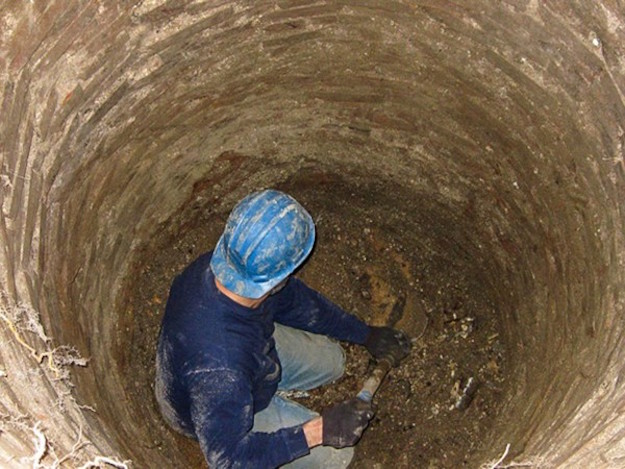MAR 26, 2015 9:06 AM PDT

# How Long to Fall Through Earth? New Answer to Old Problem

Suppose you dug a tunnel through the center of Earth, jumped in, and let gravity pull you through. How long would it take you to reach the other side of the planet? For decades, physics students have been asked to calculate that time and have been taught that the correct answer is 42 minutes. Now, a more realistic analysis has lopped 4 minutes off that estimate.

"This is the kind of paper we love," says David Jackson, a physicist at Dickinson College in Carlisle, Pennsylvania, and editor of the American Journal of Physics, a publication of the American Association of Physics Teachers. The new calculation, which appears in the March issue of the journal, doesn't merely add more detail about the structure of Earth, he notes. It also explains why you can replace one overly simple assumption with another equally crude one and still get a much more accurate answer. "That's what makes this so much fun to think about," Jackson says.The gravity tunnel problem is a staple of introductory physics courses because it simultaneously demonstrates both striking features of Isaac Newton's law of gravity and a common but very important type of cyclical motion. To solve it, students must calculate how the force of gravity on an object changes as the thing falls through the tunnel.

Here's where the usual unrealistic assumption enters. Students assume that, like a billiard ball, Earth has the same density throughout: roughly 5500 kilograms per cubic meter. In that case, the strength of the gravitational force pulling you toward Earth's center varies in proportion to the distance from the center. That's because as you descend through the tunnel, the amount of mass at an altitude lower than yours decreases, whereas the mass at an altitude higher than yours no longer has any effect on you-as students calculate using a bit of mathematics called the shell theorem.

Because the force pulling you toward the center is proportional to the distance from the center, you zip back and forth through Earth exactly as a weight on a spring bobs up or down or a pendulum swings back and forth. The gravity tunnel problem is used in teaching precisely because it produces such simple harmonic motion.

In reality, of course, Earth does not have a uniform density, but has a less dense crust and mantle and a more dense core. So Alexander Klotz, a graduate student in physics at McGill University in Montreal, Canada, started thinking about what a more realistic analysis would yield. Klotz says he isn't sure why he started mulling over the problem. But he sometimes answers physics questions on the website reddit. "I've sort of engaged in a bit of educational outreach," Klotz says, "and that [question] comes up quite a lot."

To get a more realistic mass distribution for Earth, he relied on the Preliminary Reference Earth Model, which is based on seismic data. It traces Earth's density from about less than 1000 kilograms per cubic meter at the surface to roughly 13,000 kilograms per cubic meter at the center of the core 6371 kilometers below, including a dramatic jump at the edge of the outer core, 3500 kilometers from the center. Solving the problem numerically, Klotz found that an object should fall through Earth in 38 minutes and 11 seconds, instead of the 42 minutes and 12 seconds predicted assuming a uniform planet.

Curiously, Klotz found that he got almost exactly the same answer-38 minutes flat-if he simply assumed that the force of gravity remained constant and equal to the value at the surface as an object plummeted toward the center. Such a constant force would require a different density distribution, one that increases steadily as the distance to Earth's center falls-so that when the distance to the center is halved, the density doubles-and peaks to infinity at the center. (The real distribution simply plateaus in the core, and the force of gravity diminishes to zero at the center of the core.)

So why does the constant-force approximation work so well? Because of the mass distribution in Earth, the force of gravity remains roughly constant-and actually increases slightly-down to the outer core, Klotz explains. From there on in, the force of gravity falls with distance much as in the original problem. But by that point, the object is moving so fast that it actually spends very little time passing through the core, where the constant-force approximation is obviously wrong. So in the end, the approximation is good enough.

It's that surprising explanation that makes the new analysis gratifying, Jackson says. "The classic problem needs to stay," he says. "But this is a nice addition to the classic problem." For his part, Klotz says that, in this day of big science, his experience shows that "with the right idea it's still possible to make, not a monumental discovery, but an incremental one."

(Source: Science magazine)